# SAT Math Multiple Choice Question 290: Answer and Explanation

### Test Information

Question: 290

5.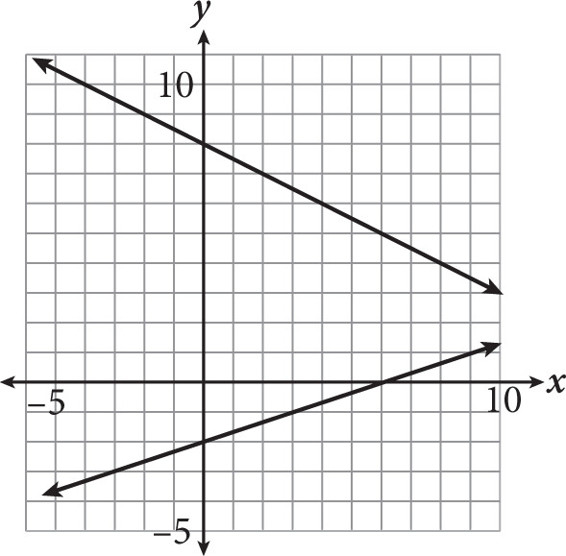What is the solution to the system of equations shown in the graph?

• A. (11, 1.5)
• B. (12, 2)
• C. (13, 2.5)
• D. (14, 2.75)

Explanation:

B

Difficulty: Medium

Category: Heart of Algebra / Systems of Linear Equations

Strategic Advice: Don't try to extend the graphs in a question like this. The answers are very close together, and it would be easy to make a mistake. Instead, think algebraically-the solution to a system of equations is the ordered pair that satisfies both equations. Thus, you need to find the equation of each line and solve the system using substitution.

Getting to the Answer: Start with the top line. Its y-intercept is 8, and it falls 1 unit for every 2 units it runs, so its slope is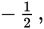making the equation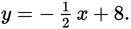The bottom line has a y-intercept of -2, and it rises 1 unit for every 3 units is runs, so its slope is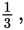making its equation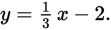Set the two equations equal to one another. Multiply everything by the common denominator, 6, to clear the fractions. Then perform inverse operations to solve for x: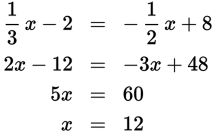You do not need to substitute 12 for x and solve for y because there is only one answer with an x-coordinate of 12, which means (B) must be correct.